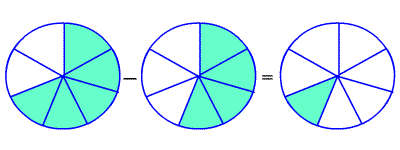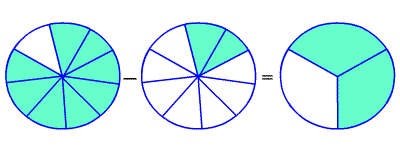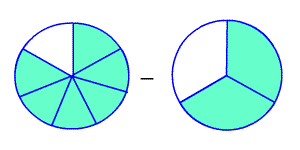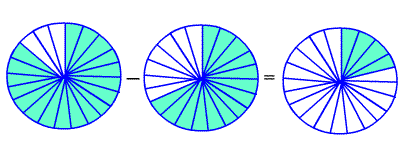# Subtracting Fractions

When subtracting fractions, the first thing to check is if the denominators are the same.

## Subtracting Fractions with Like Denominators

Fractions with the same denominators are called like fractions.

To subtract fractions with like denominators, subtract the numerators , and write the difference over the denominator.

$\frac{5}{7}-\frac{4}{7}=\frac{1}{7}$Example :

Find $\frac{4}{5}-\frac{2}{5}$ .

Since the denominators are the same, subtract the numerators.

$=\frac{4\text{\hspace{0.17em}}-\text{\hspace{0.17em}}2}{5}$

$=\frac{2}{5}$

You may get an answer which is not in lowest terms , even if the fractions you were adding and subtracting both were. In this case, you have to reduce the fraction .

$\frac{8}{9}-\frac{2}{9}=\frac{6}{9}=\frac{6\text{\hspace{0.17em}}÷\text{\hspace{0.17em}}3}{9\text{\hspace{0.17em}}÷\text{\hspace{0.17em}}3}=\frac{2}{3}$## Subtracting Fractions with Unlike Denominators

If the denominators are not the same, then you have to use equivalent fractions which do have a common denominator . To do this, you need to find the least common multiple (LCM) of the two denominators.

To subtract fractions with unlike denominators, rename the fractions with a common denominator. Then subtract and simplify.

For example, suppose you want to add:

$\frac{6}{7}-\frac{2}{3}$The LCM of $3$ and $11$ is $33$ . So, we need to find fractions equivalent to $\frac{6}{7}$ and $\frac{2}{3}$ which have $21$ in the denominator. Multiply the numerator and denominator of $\frac{6}{7}$ by $3$ , and multiply the numerator and denominator of $\frac{2}{3}$ by $7$ .

$\frac{6\text{\hspace{0.17em}}×\text{\hspace{0.17em}}3}{7\text{\hspace{0.17em}}×\text{\hspace{0.17em}}3}-\frac{2\text{\hspace{0.17em}}×\text{\hspace{0.17em}}7}{3\text{\hspace{0.17em}}×\text{\hspace{0.17em}}7}=\frac{18}{21}-\frac{14}{21}$

Now we have like denominators, and we can subtract as described above.

$=\frac{4}{21}$